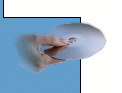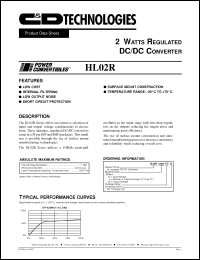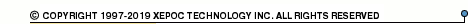More than478 253 registered clientsHL02R05D12 series datasheets. Manufacturer: CANDD.

 HL02R05D12Y Dual 2 Watt regulated DC/DC converter. Nom.input voltage 5Vdc, rated output voltage +-12Vdc, rated output current +-83mA. in 24-pin DIP package. Operational temperature range from -40°C to 85°C. Datasheet*) HL02R05D12YH Dual 2 Watt regulated DC/DC converter. Nom.input voltage 5Vdc, rated output voltage +-12Vdc, rated output current +-83mA. in 24-pin DIP package. Operational temperature range from -40°C to 85°C. Datasheet*) HL02R05D12Z Dual 2 Watt regulated DC/DC converter. Nom.input voltage 5Vdc, rated output voltage +-12Vdc, rated output current +-83mA. in 24-pin SMD package. Operational temperature range from -40°C to 85°C. Datasheet*) HL02R05D15Y Dual 2 Watt regulated DC/DC converter. Nom.input voltage 5Vdc, rated output voltage +-15Vdc, rated output current +-67mA. in 24-pin DIP package. Operational temperature range from -40°C to 85°C. Datasheet*) HL02R05D15YH Dual 2 Watt regulated DC/DC converter. Nom.input voltage 5Vdc, rated output voltage +-15Vdc, rated output current +-67mA. in 24-pin DIP package. Operational temperature range from -40°C to 85°C. Datasheet*) HL02R05D15Z Dual 2 Watt regulated DC/DC converter. Nom.input voltage 5Vdc, rated output voltage +-15Vdc, rated output current +-67mA. in 24-pin SMD package. Operational temperature range from -40°C to 85°C. Datasheet*) HL02R05S05Y Single 2 Watt regulated DC/DC converter. Nom.input voltage 5Vdc, rated output voltage 5Vdc, rated output current 400mA. in 24-pin DIP package. Operational temperature range from -40°C to 85°C. Datasheet*) HL02R05S05YH Single 2 Watt regulated DC/DC converter. Nom.input voltage 5Vdc, rated output voltage 5Vdc, rated output current 400mA. in 24-pin DIP package. Operational temperature range from -40°C to 85°C. Datasheet*) HL02R05S05Z Single 2 Watt regulated DC/DC converter. Nom.input voltage 5Vdc, rated output voltage 5Vdc, rated output current 400mA. in 24-pin SMD package. Operational temperature range from -40°C to 85°C. Datasheet*) HL02R05S12Y Single 2 Watt regulated DC/DC converter. Nom.input voltage 5Vdc, rated output voltage 12Vdc, rated output current 166mA. in 24-pin DIP package. Operational temperature range from -40°C to 85°C. Datasheet*) HL02R05S12YH Single 2 Watt regulated DC/DC converter. Nom.input voltage 5Vdc, rated output voltage 12Vdc, rated output current 166mA. in 24-pin DIP package. Operational temperature range from -40°C to 85°C. Datasheet*) HL02R05S12Z Single 2 Watt regulated DC/DC converter. Nom.input voltage 5Vdc, rated output voltage 12Vdc, rated output current 166mA. in 24-pin SMD package. Operational temperature range from -40°C to 85°C. Datasheet*) HL02R05S15Y Single 2 Watt regulated DC/DC converter. Nom.input voltage 5Vdc, rated output voltage 15Vdc, rated output current 134mA. in 24-pin DIP package. Operational temperature range from -40°C to 85°C. Datasheet*) HL02R05S15YH Single 2 Watt regulated DC/DC converter. Nom.input voltage 5Vdc, rated output voltage 15Vdc, rated output current 134mA. in 24-pin DIP package. Operational temperature range from -40°C to 85°C. Datasheet*) HL02R05S15Z Single 2 Watt regulated DC/DC converter. Nom.input voltage 5Vdc, rated output voltage 15Vdc, rated output current 134mA. in 24-pin SMD package. Operational temperature range from -40°C to 85°C. Datasheet*) HL02R12D12Y Dual 2 Watt regulated DC/DC converter. Nom.input voltage 12Vdc, rated output voltage +-12Vdc, rated output current +-83mA. in 24-pin DIP package. Operational temperature range from -40°C to 85°C. Datasheet*) HL02R12D12YH Dual 2 Watt regulated DC/DC converter. Nom.input voltage 12Vdc, rated output voltage +-12Vdc, rated output current +-83mA. in 24-pin DIP package. Operational temperature range from -40°C to 85°C. Datasheet*) HL02R12D12Z Dual 2 Watt regulated DC/DC converter. Nom.input voltage 12Vdc, rated output voltage +-12Vdc, rated output current +-83mA. in 24-pin SMD package. Operational temperature range from -40°C to 85°C. Datasheet*) HL02R12D15Y Dual 2 Watt regulated DC/DC converter. Nom.input voltage 12Vdc, rated output voltage +-15Vdc, rated output current +-67mA. in 24-pin DIP package. Operational temperature range from -40°C to 85°C. Datasheet*) HL02R12D15YH Dual 2 Watt regulated DC/DC converter. Nom.input voltage 12Vdc, rated output voltage +-15Vdc, rated output current +-67mA. in 24-pin DIP package. Operational temperature range from -40°C to 85°C. Datasheet*) HL02R12D15Z Dual 2 Watt regulated DC/DC converter. Nom.input voltage 12Vdc, rated output voltage +-15Vdc, rated output current +67mA. in 24-pin SMD package. Operational temperature range from -40°C to 85°C. Datasheet*) HL02R12S05Y Single 2 Watt regulated DC/DC converter. Nom.input voltage 12Vdc, rated output voltage 5Vdc, rated output current 400mA. in 24-pin DIP package. Operational temperature range from -40°C to 85°C. Datasheet*) HL02R12S05YH Single 2 Watt regulated DC/DC converter. Nom.input voltage 12Vdc, rated output voltage 5Vdc, rated output current 400mA. in 24-pin DIP package. Operational temperature range from -40°C to 85°C. Datasheet*) HL02R12S05Z Single 2 Watt regulated DC/DC converter. Nom.input voltage 12Vdc, rated output voltage 5Vdc, rated output current 400mA. in 24-pin SMD package. Operational temperature range from -40°C to 85°C. Datasheet*) HL02R12S12Y Single 2 Watt regulated DC/DC converter. Nom.input voltage 12Vdc, rated output voltage 12Vdc, rated output current 166mA. in 24-pin DIP package. Operational temperature range from -40°C to 85°C. Datasheet*) HL02R12S12YH Single 2 Watt regulated DC/DC converter. Nom.input voltage 12Vdc, rated output voltage 12Vdc, rated output current 166mA. in 24-pin DIP package. Operational temperature range from -40°C to 85°C. Datasheet*) HL02R12S12Z Single 2 Watt regulated DC/DC converter. Nom.input voltage 12Vdc, rated output voltage 12Vdc, rated output current 166mA. in 24-pin SMD package. Operational temperature range from -40°C to 85°C. Datasheet*) HL02R12S15Y Single 2 Watt regulated DC/DC converter. Nom.input voltage 12Vdc, rated output voltage 15Vdc, rated output current 134mA. in 24-pin DIP package. Operational temperature range from -40°C to 85°C. Datasheet*) HL02R12S15YH Single 2 Watt regulated DC/DC converter. Nom.input voltage 12Vdc, rated output voltage 15Vdc, rated output current 134mA. in 24-pin DIP package. Operational temperature range from -40°C to 85°C. Datasheet*) HL02R12S15Z Single 2 Watt regulated DC/DC converter. Nom.input voltage 12Vdc, rated output voltage 15Vdc, rated output current 134mA. in 24-pin SMD package. Operational temperature range from -40°C to 85°C. Datasheet*) HL02R15D12Y Dual 2 Watt regulated DC/DC converter. Nom.input voltage 15Vdc, rated output voltage +-12Vdc, rated output current +-83mA. in 24-pin DIP package. Operational temperature range from -40°C to 85°C. Datasheet*) HL02R15D12YH Dual 2 Watt regulated DC/DC converter. Nom.input voltage 15Vdc, rated output voltage +-12Vdc, rated output current +-83mA. in 24-pin DIP package. Operational temperature range from -40°C to 85°C. Datasheet*) HL02R15D12Z Dual 2 Watt regulated DC/DC converter. Nom.input voltage 15Vdc, rated output voltage +-12Vdc, rated output current +-83mA. in 24-pin SMD package. Operational temperature range from -40°C to 85°C. Datasheet*) HL02R15D15Y Dual 2 Watt regulated DC/DC converter. Nom.input voltage 15Vdc, rated output voltage +-15Vdc, rated output current +-67mA. in 24-pin DIP package. Operational temperature range from -40°C to 85°C. Datasheet*) HL02R15D15YH Dual 2 Watt regulated DC/DC converter. Nom.input voltage 15Vdc, rated output voltage +-15Vdc, rated output current +-67mA. in 24-pin DIP package. Operational temperature range from -40°C to 85°C. Datasheet*) HL02R15D15Z Dual 2 Watt regulated DC/DC converter. Nom.input voltage 15Vdc, rated output voltage +-15Vdc, rated output current +-67mA. in 24-pin SMD package. Operational temperature range from -40°C to 85°C. Datasheet*) HL02R15S05Y Single 2 Watt regulated DC/DC converter. Nom.input voltage 15Vdc, rated output voltage 5Vdc, rated output current 400mA. in 24-pin DIP package. Operational temperature range from -40°C to 85°C. Datasheet*) HL02R15S05YH Single 2 Watt regulated DC/DC converter. Nom.input voltage 15Vdc, rated output voltage 5Vdc, rated output current 400mA. in 24-pin DIP package. Operational temperature range from -40°C to 85°C. Datasheet*) HL02R15S05Z Single 2 Watt regulated DC/DC converter. Nom.input voltage 15Vdc, rated output voltage 5Vdc, rated output current 400mA. in 24-pin SMD package. Operational temperature range from -40°C to 85°C. Datasheet*) HL02R15S12Y Single 2 Watt regulated DC/DC converter. Nom.input voltage 15Vdc, rated output voltage 12Vdc, rated output current 166mA. in 24-pin DIP package. Operational temperature range from -40°C to 85°C. Datasheet*) HL02R15S12YH Single 2 Watt regulated DC/DC converter. Nom.input voltage 15Vdc, rated output voltage 12Vdc, rated output current 166mA. in 24-pin DIP package. Operational temperature range from -40°C to 85°C. Datasheet*) HL02R15S12Z Single 2 Watt regulated DC/DC converter. Nom.input voltage 15Vdc, rated output voltage 12Vdc, rated output current 166mA. in 24-pin SMD package. Operational temperature range from -40°C to 85°C. Datasheet*) HL02R15S15Y Single 2 Watt regulated DC/DC converter. Nom.input voltage 15Vdc, rated output voltage 15Vdc, rated output current 134mA. in 24-pin DIP package. Operational temperature range from -40°C to 85°C. Datasheet*) HL02R15S15YH Single 2 Watt regulated DC/DC converter. Nom.input voltage 15Vdc, rated output voltage 15Vdc, rated output current 134mA. in 24-pin DIP package. Operational temperature range from -40°C to 85°C. Datasheet*) HL02R15S15Z Single 2 Watt regulated DC/DC converter. Nom.input voltage 15Vdc, rated output voltage 15Vdc, rated output current 134mA. in 24-pin SMD package. Operational temperature range from -40°C to 85°C. Datasheet*) HL02R24D12Y Dual 2 Watt regulated DC/DC converter. Nom.input voltage 24Vdc, rated output voltage +-12Vdc, rated output current +-83mA. in 24-pin DIP package. Operational temperature range from -40°C to 85°C. Datasheet*) HL02R24D12YH Dual 2 Watt regulated DC/DC converter. Nom.input voltage 24Vdc, rated output voltage +-12Vdc, rated output current +-83mA. in 24-pin DIP package. Operational temperature range from -40°C to 85°C. Datasheet*) HL02R24D12Z Dual 2 Watt regulated DC/DC converter. Nom.input voltage 24Vdc, rated output voltage +-12Vdc, rated output current +-83mA. in 24-pin SMD package. Operational temperature range from -40°C to 85°C. Datasheet*) HL02R24D15Y Dual 2 Watt regulated DC/DC converter. Nom.input voltage 24Vdc, rated output voltage +-15Vdc, rated output current +-67mA. in 24-pin DIP package. Operational temperature range from -40°C to 85°C. Datasheet*) HL02R24D15YH Dual 2 Watt regulated DC/DC converter. Nom.input voltage 24Vdc, rated output voltage +-15Vdc, rated output current +-67mA. in 24-pin DIP package. Operational temperature range from -40°C to 85°C. Datasheet*) HL02R24D15Z Dual 2 Watt regulated DC/DC converter. Nom.input voltage 24Vdc, rated output voltage +-15Vdc, rated output current +-67mA. in 24-pin SMD package. Operational temperature range from -40°C to 85°C. Datasheet*) HL02R24S05Y Single 2 Watt regulated DC/DC converter. Nom.input voltage 24Vdc, rated output voltage 5Vdc, rated output current 400mA. in 24-pin DIP package. Operational temperature range from -40°C to 85°C. Datasheet*) HL02R24S05YH Single 2 Watt regulated DC/DC converter. Nom.input voltage 24Vdc, rated output voltage 5Vdc, rated output current 400mA. in 24-pin DIP package. Operational temperature range from -40°C to 85°C. Datasheet*) HL02R24S05Z Single 2 Watt regulated DC/DC converter. Nom.input voltage 24Vdc, rated output voltage 5Vdc, rated output current 400mA. in 24-pin SMD package. Operational temperature range from -40°C to 85°C. Datasheet*) HL02R24S12Y Single 2 Watt regulated DC/DC converter. Nom.input voltage 24Vdc, rated output voltage 12Vdc, rated output current 166mA. in 24-pin DIP package. Operational temperature range from -40°C to 85°C. Datasheet*) HL02R24S12YH Single 2 Watt regulated DC/DC converter. Nom.input voltage 24Vdc, rated output voltage 12Vdc, rated output current 166mA. in 24-pin DIP package. Operational temperature range from -40°C to 85°C. Datasheet*) HL02R24S12Z Single 2 Watt regulated DC/DC converter. Nom.input voltage 24Vdc, rated output voltage 12Vdc, rated output current 166mA. in 24-pin SMD package. Operational temperature range from -40°C to 85°C. Datasheet*) HL02R24S15Y Single 2 Watt regulated DC/DC converter. Nom.input voltage 24Vdc, rated output voltage 15Vdc, rated output current 134mA. in 24-pin DIP package. Operational temperature range from -40°C to 85°C. Datasheet*) HL02R24S15YH Single 2 Watt regulated DC/DC converter. Nom.input voltage 24Vdc, rated output voltage 15Vdc, rated output current 134mA. in 24-pin DIP package. Operational temperature range from -40°C to 85°C. Datasheet*) HL02R24S15Z Single 2 Watt regulated DC/DC converter. Nom.input voltage 24Vdc, rated output voltage 15Vdc, rated output current 134mA. in 24-pin SMD package. Operational temperature range from -40°C to 85°C. Datasheet*) HL02R 2 WATT REGULATED DC/DC CONVERTER Datasheet*) HL02R05D12 2 WATT REGULATED DC/DC CONVERTER Datasheet*) HL02R05D15 2 WATT REGULATED DC/DC CONVERTER Datasheet*) HL02R05S05 2 WATT REGULATED DC/DC CONVERTER Datasheet*) HL02R05S12 2 WATT REGULATED DC/DC CONVERTER Datasheet*) HL02R05S15 2 WATT REGULATED DC/DC CONVERTER Datasheet*) HL02R12D12 2 WATT REGULATED DC/DC CONVERTER Datasheet*) HL02R12D15 2 WATT REGULATED DC/DC CONVERTER Datasheet*) HL02R12S05 2 WATT REGULATED DC/DC CONVERTER Datasheet*) HL02R12S12 2 WATT REGULATED DC/DC CONVERTER Datasheet*) HL02R12S15 2 WATT REGULATED DC/DC CONVERTER Datasheet*) HL02R15D12 2 WATT REGULATED DC/DC CONVERTER Datasheet*) HL02R15D15 2 WATT REGULATED DC/DC CONVERTER Datasheet*) HL02R15S05 2 WATT REGULATED DC/DC CONVERTER Datasheet*) HL02R15S12 2 WATT REGULATED DC/DC CONVERTER Datasheet*) HL02R15S15 2 WATT REGULATED DC/DC CONVERTER Datasheet*) HL02R24D12 2 WATT REGULATED DC/DC CONVERTER Datasheet*) HL02R24D15 2 WATT REGULATED DC/DC CONVERTER Datasheet*) HL02R24S05 2 WATT REGULATED DC/DC CONVERTER Datasheet*) HL02R24S12 2 WATT REGULATED DC/DC CONVERTER Datasheet*) HL02R24S15 2 WATT REGULATED DC/DC CONVERTER Datasheet*)